# Search

About 245 Search Results Matching Types of Worksheet, Worksheet Section, Generator, Generator Section, Subjects matching Math, Grades matching 3rd Grade, Similar to Reading a Protractor Worksheet 2, Page 3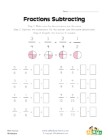## Simple Fraction Subtraction Worksheet 2

This worksheet has 8 simple subtracting like fract...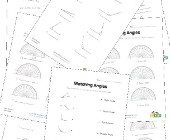## Angles Worksheets

Help kids learn about angles with this collection ...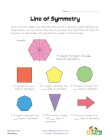## Lines of Symmetry Worksheet 2

Look at the shapes, count and write down how many ...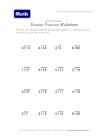## Division with Remainders Worksheet 2

16 division problems that include remainders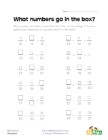## Missing Numbers in Fraction Equations Worksheet 2

Fill in the missing numbers in each of the like fr...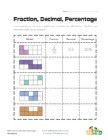## Fractions, Decimals and Percentages Worksheet 2

Use the model to figure out the fraction, decimal ...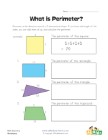## Perimeter Worksheet 1

Add up the length of the sides of each shape to fi...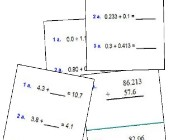Help kids practice decimal addition with the follo...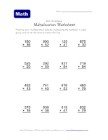## Multiple Digit Multiplication Worksheet 1

3 digits by 2 digits multiplication problems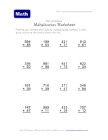## Multiple Digit Multiplication Worksheet 3

3 digits multiplied by 2 digits problems